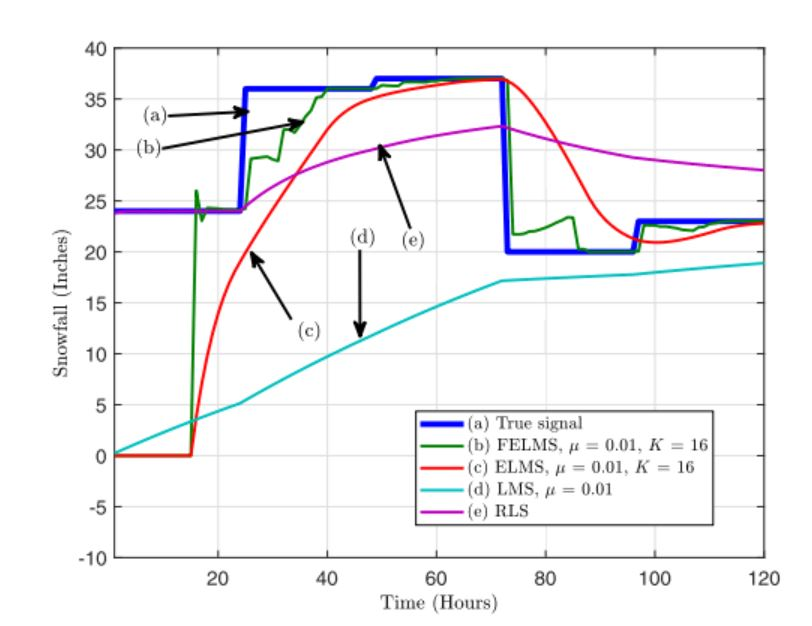# Paper: Efficient estimation of graph signals with adaptive sampling

We propose two new least mean squares (LMS)-based algorithms for adaptive estimation of graph signals that improve the convergence speed of the LMS algorithm while preserving its low computational complexity.

The first algorithm, named extended least mean squares (ELMS), extends the LMS algorithm by virtue of reusing the signal vectors of previous iterations alongside the signal available at the current iteration. Utilizing the previous signal vectors accelerates the convergence of the ELMS algorithm at the expense of higher steady-state error compared to the LMS algorithm.

To further improve the performance, we propose the fast ELMS (FELMS) algorithm in which the influence of the signal vectors of previous iterations is controlled by optimizing the gradient of the mean-square deviation (GMSD). The FELMS algorithm converges faster than the ELMS algorithm and has steady-state errors comparable to that of the LMS algorithm.

We analyze the mean-square performance of ELMS and FELMS algorithms theoretically and derive the respective convergence conditions as well as the predicted MSD values. In addition, we present an adaptive sampling strategy in which the sampling probability of each node is changed according to the GMSD of the node.

Computer simulations using both synthetic and real data validate the theoretical results and demonstrate the merits of the proposed algorithms.Fig. 24. True snowfall and its estimates versus time at a randomly chosen unobserved station, for different algorithms

M. J. Ahmadi, R. Arablouei and R. Abdolee, “Efficient Estimation of Graph Signals With Adaptive Sampling,” in IEEE Transactions on Signal Processing, vol. 68, pp. 3808-3823, 2020, doi: 10.1109/TSP.2020.3002607.